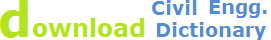triangulation data, definition of triangulation, surveying research triangulation

# Steps in carrying out Triangulation work - How to do triangulation

Triangulation work is carried out in following step.

1. Reconaissance
2. Erection of Signals & Towers
3. Measurement of Horizontal Angles
4. Astronomical Observations Necessary to Determine the True Meridian and the Absolute Positions of the Stations
5. Measurement of Baseline
7. Computations of Legths of each side of each8. Computations of the Latitude and Longitude of ST

## 1. Reconaissance:

In geodetic Surveying, reconaissance consists of: (See also: Reconaissance in Transportation & Highway Engineering )

1. Examination of the country to be surveyed.
2. Selection of most favorable sides for base lines
3. Selection of suitable positions ofstation
4. Determination of indivisibility of station

## 2. Selection of Station:

The selection of station is based upon the following consideration.

1. The stations should be clearly visible from each other. For this purpose highest commanding positions such as top of hills or mountains is selected.
2. They should form well shaped triangles
3. They should be easily accessible
4. They should be useful for detail survey
5. Thy should be so fixed that the length of sight is not too short or too long

## 3. Intervisibility and Hights of Stations:

For indivisibility of two stations they should be fixed on highest available ground. Such as mountain peaks rides or top of hills when the distance b/t the two stations is great and the difference in elevation b/t them is small then it is necessary to raise both the instrument and signal to overcome the curvature of the earth and to clear all the intervening obstruction.

The height of both the instrument and signal above the ground depends upon.

1. Distance between the stations
2. Relative elevations of stations
3. The profile of intervening ground

### 3. 1. Distance between stations:

If the intervening ground is free any obstruction, the distance of a visible horizon form a station of known elves above datum as well as the elves of the signal while may be just visible at a given distance can be determine from the formula.Where

H = ht. of station above a datum
D = Dist from the station to point of tangent
R = mean radius of earth
M = co-efficient of refraction (0.07 for sight over land and 0.08 for sights over water)

D1 and R being expressed in same units. Alternatively, if ‘h’ is in meter and D1 is in kilometer then

H (m) = 0.0673 D12 (km) ==> (A)

Or

H (ft) = 0.574 D1 (miles) ==> (B)

D1 and D2 can be determined and dist b/t two stations will be (D1+ D2)

### 3. 2. Relative elevations of stations:

A and B are the two stations

D = dist b/t A and B in km
Ha = known elev of ‘A’ above a datum
H = required elev of ‘B’ above a datum
D1 = distance (km) of ‘A’ from pt of tangency (p)
D2 = distance (km) of ‘B’

Dist D1 can be calculated. Hence the required elev, h = 0.0673 D2

Ha = 0.0673 D12   or       h = 0.0574 D22 (miles)OrNow D2 = D - D1

Height or signal / Tower/ scaffold a B = Elev of datum + h - R.L of st B elev of line sight

NOTE:

The line of sight should not be near the surface of ground at pt of tangency on account of strata of disturbed air and should be kept at least 2m (61) above the ground permeably 3m (1D) and this allowance (clearance) should be made in deterring the heights of stations.

### 3. 3. Profile of intervening ground:

If the peaks in the intervening ground are likely to obstruct, the line of sight, their elevations and locations must be determined.

#### Procedure:

The elevation of line of sights at the respective points can be computed and the results compared with the ground elevation at those points to determine weather the line of sight clears all the intervening obstruction.Site Map | Civil Engineering Training | Contact Us || © 2014 WebTechTix